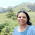## Dec 30, 2015

### How to show a friendly time difference in javascript

This is a short reusable coding snippet that could save you some programming time. I was creating one for myself, and later figured out I would put it up here on my blog as well, in case someone needs it.

The objective is to take in a date as input and then provide the date difference in a meaningful way. For example, if the difference is few seconds, it should say "A moment ago", instead of 17.563 seconds. Also it makes sense to say "Yesterday", instead of "27.75 hours ago". The script below is created  using such assumption.

```//t is a standard ISO date string like '2015-12-29T19:07:41Z'

function getFriendlyTime(t)
{
var diff = Date.now() - Date.parse(t);
var seconds = 1000, minutes = 1000 * 60, hours = 1000 * 60 * 60, days = 1000 * 60 * 60 * 24, weeks = 1000 * 60 * 60 * 24 * 7, months = 1000 * 60 * 60 * 24 * 30, year = 1000 * 60 * 60 * 24 * 365;
if(diff < 2 * minutes) return "A moment ago";
if(diff < hours) return Math.floor(diff/minutes) + " mins ago";
if(diff < days) return (Math.floor(diff/hours)==1)?"an hour ago":Math.floor(diff/hours) + " hrs ago";
if(diff < weeks) return (Math.floor(diff/days)==1)?"yesterday":Math.floor(diff/days) + " days ago";
if(diff < months) return (Math.floor(diff/weeks)==1)?"last week":Math.floor(diff/weeks) + " weeks ago";
if(diff < year) return (Math.floor(diff/months)==1)?"last month":Math.floor(diff/months) + " months ago";
return (Math.floor(diff/year)==1)?"an year ago":Math.floor(diff/year) + " yrs ago";
}
```

#### 1 comment:

1.